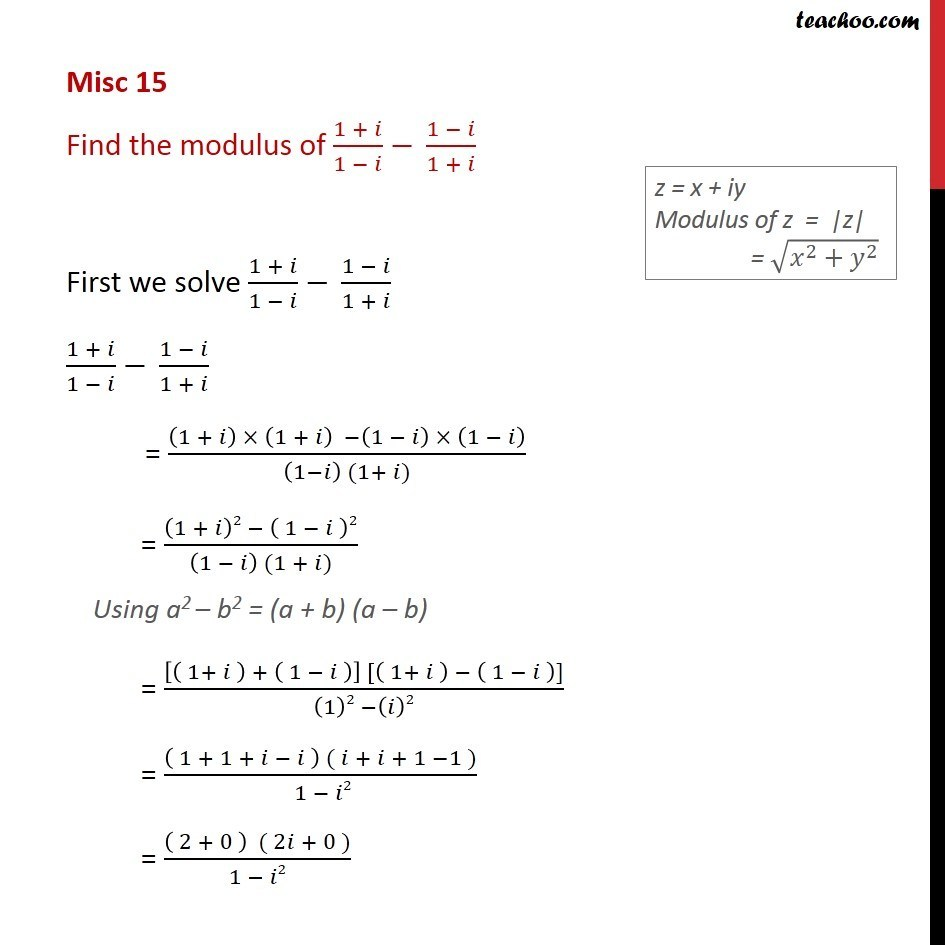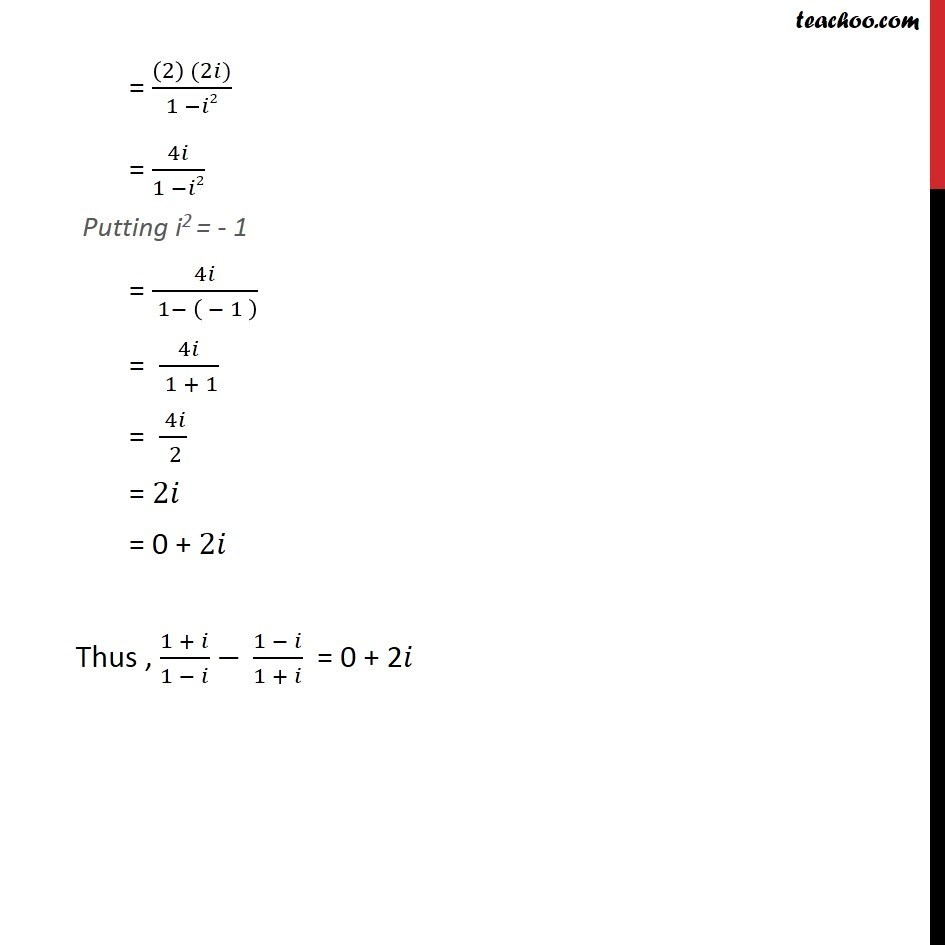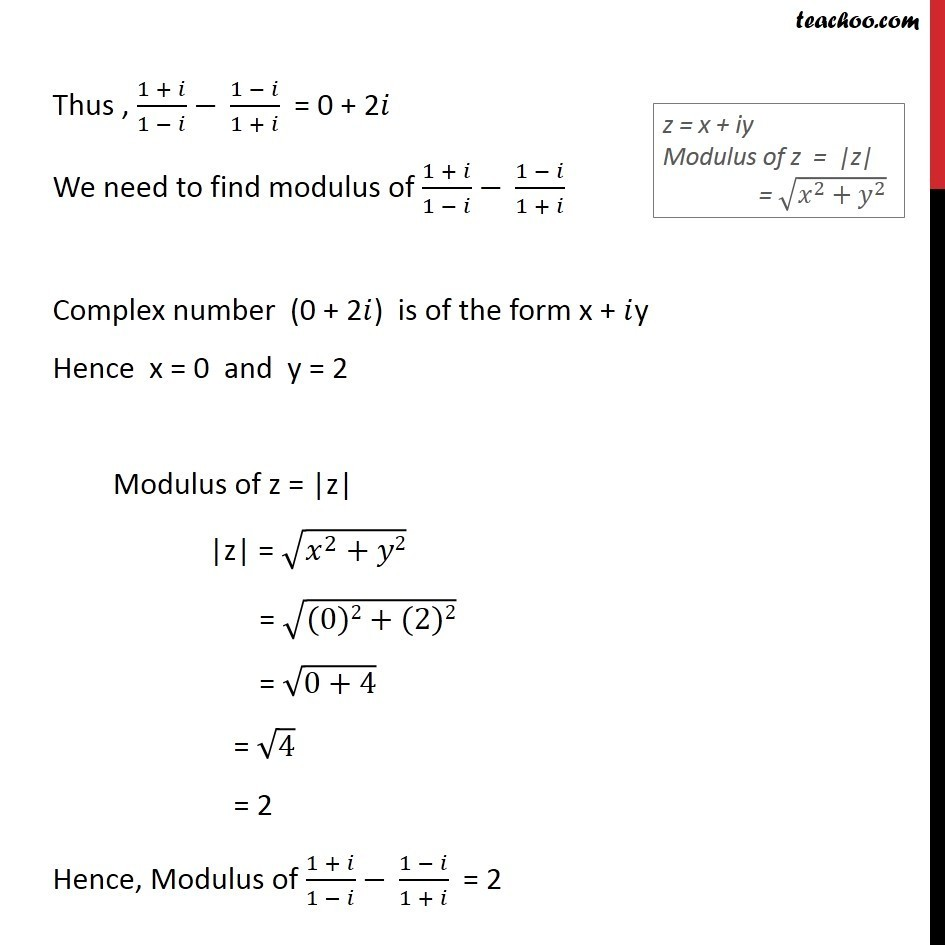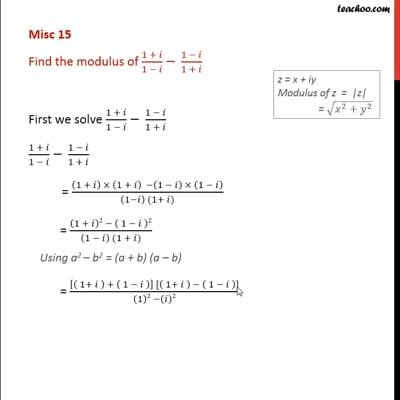Miscellaneous

Chapter 4 Class 11 Complex Numbers
Serial order wiseThis video is only available for Teachoo black users

Learn in your speed, with individual attention - Teachoo Maths 1-on-1 Class

### Transcript

Misc 9 Find the modulus of (1 + )/(1 ) (1 )/(1 + ) First we solve (1 + )/(1 ) (1 )/(1 + ) (1 + )/(1 ) (1 )/(1 + ) = ((1 + ) (1 + ) (1 ) (1 ))/((1 ) (1+ )) = ((1 + )2 ( 1 )2)/((1 ) (1 + )) Using a2 b2 = (a + b) (a b) = ([( 1+ ) + ( 1 )] [( 1+ ) ( 1 )])/((1)2 ( )2) = (( 1 + 1 + ) ( + + 1 1 ))/( 1 2) = (( 2 + 0 ) ( 2 + 0 ))/(1 2) = ((2) (2 ))/(1 2) = 4 /(1 2) Putting i2 = - 1 = 4 /( 1 ( 1 ) ) = 4 /( 1 + 1) = ( 4 )/( 2) = 2 = 0 + 2 Thus , (1 + )/(1 ) (1 )/(1 + ) = 0 + 2 Thus , (1 + )/(1 ) (1 )/(1 + ) = 0 + 2 We need to find modulus of (1 + )/(1 ) (1 )/(1 + ) Complex number (0 + 2 ) is of the form x + y Hence x = 0 and y = 2 Modulus of z = |z| |z| = ( ^2+ 2) = ((0)2+(2)2) = (0+4) = 4 = 2 Hence, Modulus of (1 + )/(1 ) (1 )/(1 + ) = 2